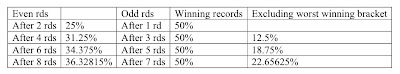## Tuesday, April 21, 2009

### Pascal's triangle and brackets

As many debate coaches and competitors already know, because teams are always power-matching within brackets against opponents with the same record, it is possible to construct a simple branching diagram to predict how many teams are in each bracket, like so:

100
50 50
25 50 25

and so on. What they may not realize is the connection to Pascal's triangle, a famous problem in mathematics:Here are the first nine rows. Each number in a row is created by adding the two numbers in the row above, the number diagonally left and the number diagonally right. The numbers in each row can easily be turned into percentages by dividing a number by the total of the row. For example, in row one, 1/1 is 100%; in row two, 1/2 is 50%; in row three, 1/4 is 25%. As you might have noticed, the total for each row is always 2^(n-1), where n is the row number. (There's also a shortcut for finding the numbers in the rows.)

Once everything has been converted into a percentage, it can be organized in a more easy-to-read chart:Of course, some of these percentages are overly precise for a debate tournament. The percentages never quite work out exactly because of pull ups caused by uneven numbered brackets, side constraints, team constraints, etc. I always use the exact percentage to multiply by the number of teams at the tournament -- but I am never surprised by +/- 1 or even 2 teams off the prediction. Still, it can be a simple way to estimate the number of teams in each bracket, especially at a very large tournament where the constraints will have less effect. I've looked at the data from large tournaments before and found that the bracket size predictions were within a percentage point. That estimation is useful to estimate the number of teams that could break:The left two columns show the percentages of teams with a winning record (excluding those with a 50-50% win-loss record) after various even numbers of rounds. The middle columns show the percentage of teams with winning records after various odd numbers of rounds -- always 50%. The right-most column shows the percentages of teams with winning records excluding the worst winning bracket (i.e., excluding 2-1s after three rounds, 3-2s after five rounds, and 4-3s after seven rounds).